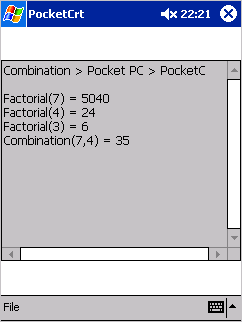Home > Pocket PC > Samples > Combination > PocketCCombination in PocketC

####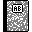Purpose

This is the PocketC version of our combination application.

####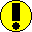Requirements

You need PocketC (see tools section).

####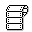Source code

Below is the .pc file.

```// Combination.pc
// Pocket PC
// PocketC

int Factorial(int n)
{
if (n <= 1)
return 1;
else return n*Factorial(n-1);
}

int Combination(int n, int p)
{
return Factorial(n)/(Factorial(n - p) * Factorial(p));
}

main()
{
int n = 0, p = 0;
char buf;

clear();
putsl("Combination > Pocket PC > PocketC");
putsl(" ");

n = gets("Value for 'n': ");
p = gets("Value for 'p': ");

putsl("Factorial(" +n +") = " +Factorial(n));
putsl("Factorial(" +p +") = " +Factorial(p));
putsl("Factorial(" +(n-p) +") = " +Factorial(n-p));
putsl("Combination(" +n +"," +p +") = " +Combination(n,p));
}
```

####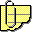Comments

Here comes PocketC again, if you've already read the Palm section of our samples. Actually, the source code is exactly the same as for Palm, except that you won't find the clauses starting with @ that are used on Palm platform to specify resources (like creator ID), and we're using putsl() instead of puts()...

This version is sensibly shorter than the other versions of combination for Pocket PC.

The core functions are located at the beginning of the .c file: Factorial(), Combination() and main().